# Time value of money

4 de Feb de 2018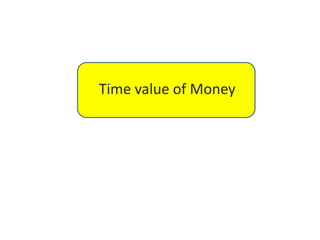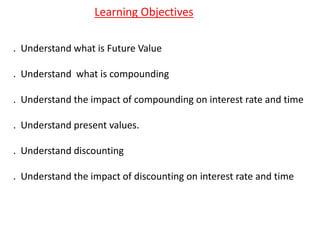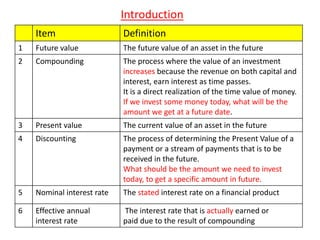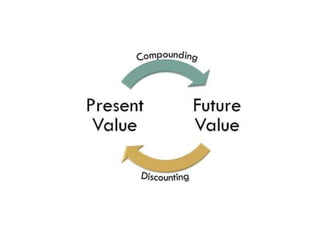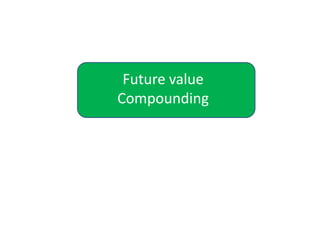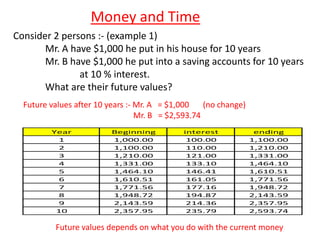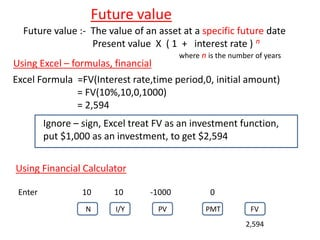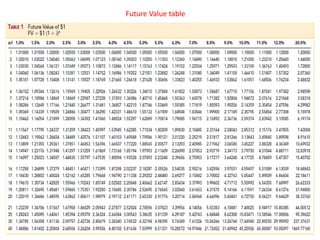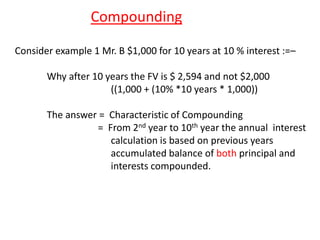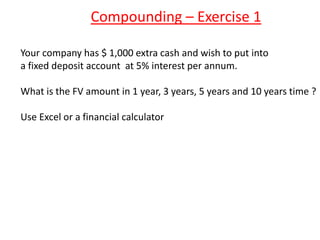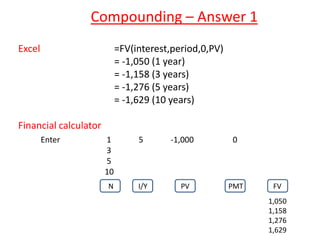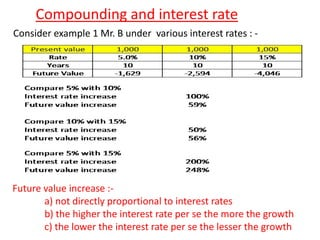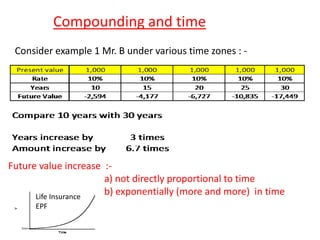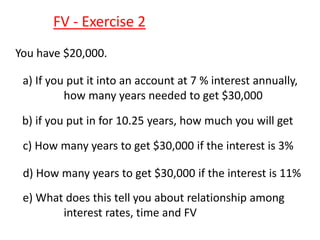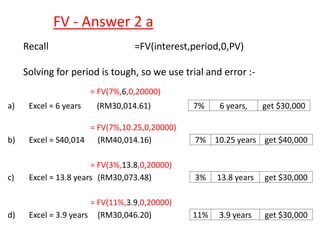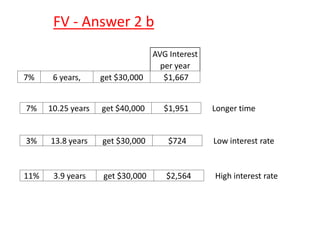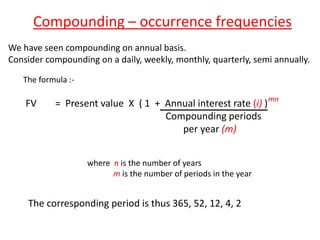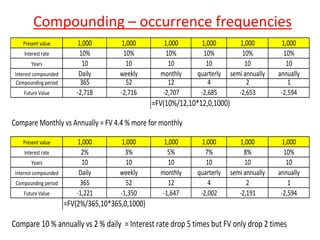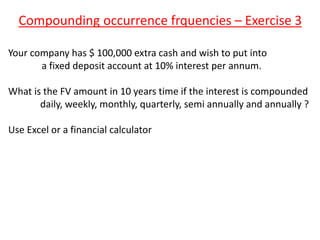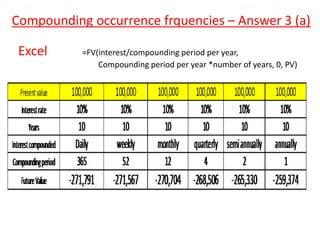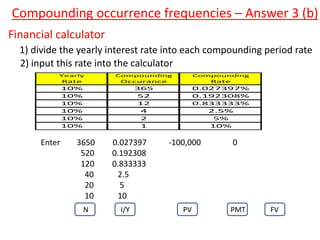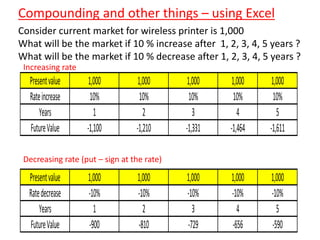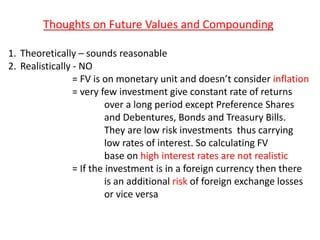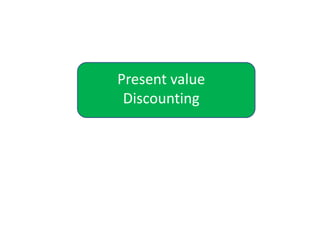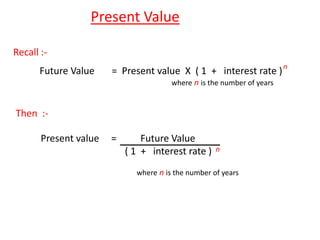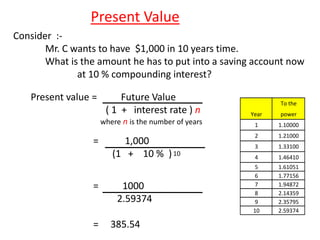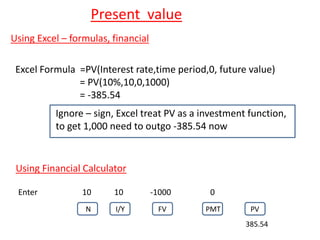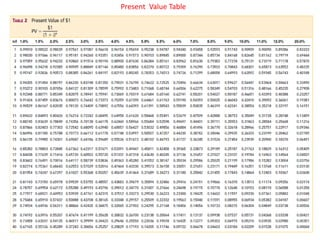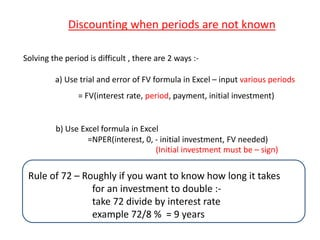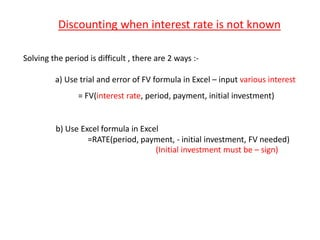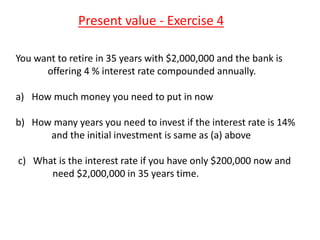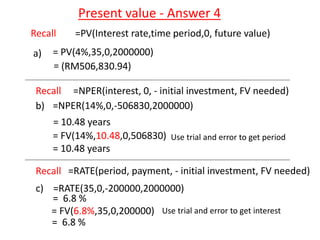1 de 32

### Time value of money

• 2. Learning Objectives . Understand what is Future Value . Understand what is compounding . Understand the impact of compounding on interest rate and time . Understand present values. . Understand discounting . Understand the impact of discounting on interest rate and time
• 3. Introduction Item Definition 1 Future value The future value of an asset in the future 2 Compounding The process where the value of an investment increases because the revenue on both capital and interest, earn interest as time passes. It is a direct realization of the time value of money. If we invest some money today, what will be the amount we get at a future date. 3 Present value The current value of an asset in the future 4 Discounting The process of determining the Present Value of a payment or a stream of payments that is to be received in the future. What should be the amount we need to invest today, to get a specific amount in future. 5 Nominal interest rate The stated interest rate on a financial product 6 Effective annual interest rate The interest rate that is actually earned or paid due to the result of compounding
• 6. Money and Time Consider 2 persons :- (example 1) Mr. A have \$1,000 he put in his house for 10 years Mr. B have \$1,000 he put into a saving accounts for 10 years at 10 % interest. What are their future values? Future values after 10 years :- Mr. A = \$1,000 (no change) Mr. B = \$2,593.74 Future values depends on what you do with the current money Year Beginning interest ending 1 1,000.00 100.00 1,100.00 2 1,100.00 110.00 1,210.00 3 1,210.00 121.00 1,331.00 4 1,331.00 133.10 1,464.10 5 1,464.10 146.41 1,610.51 6 1,610.51 161.05 1,771.56 7 1,771.56 177.16 1,948.72 8 1,948.72 194.87 2,143.59 9 2,143.59 214.36 2,357.95 10 2,357.95 235.79 2,593.74
• 7. Future value Future value :- The value of an asset at a specific future date Present value X ( 1 + interest rate ) where n is the number of years n Excel Formula =FV(Interest rate,time period,0, initial amount) = FV(10%,10,0,1000) = 2,594 Using Excel – formulas, financial Ignore – sign, Excel treat FV as an investment function, put \$1,000 as an investment, to get \$2,594 Using Financial Calculator Enter 10 10 -1000 0 N I/Y PV PMT FV 2,594
• 9. Compounding Consider example 1 Mr. B \$1,000 for 10 years at 10 % interest :=– Why after 10 years the FV is \$ 2,594 and not \$2,000 ((1,000 + (10% *10 years * 1,000)) The answer = Characteristic of Compounding = From 2nd year to 10th year the annual interest calculation is based on previous years accumulated balance of both principal and interests compounded.
• 10. Compounding – Exercise 1 Your company has \$ 1,000 extra cash and wish to put into a fixed deposit account at 5% interest per annum. What is the FV amount in 1 year, 3 years, 5 years and 10 years time ? Use Excel or a financial calculator
• 11. Compounding – Answer 1 Excel =FV(interest,period,0,PV) = -1,050 (1 year) = -1,158 (3 years) = -1,276 (5 years) = -1,629 (10 years) Financial calculator Enter 1 5 -1,000 0 3 5 10 N I/Y PV PMT FV 1,050 1,158 1,276 1,629
• 12. Compounding and interest rate Consider example 1 Mr. B under various interest rates : - Future value increase :- a) not directly proportional to interest rates b) the higher the interest rate per se the more the growth c) the lower the interest rate per se the lesser the growth
• 13. Compounding and time Consider example 1 Mr. B under various time zones : - Future value increase :- a) not directly proportional to time b) exponentially (more and more) in timeLife Insurance EPF
• 14. FV - Exercise 2 You have \$20,000. a) If you put it into an account at 7 % interest annually, how many years needed to get \$30,000 b) if you put in for 10.25 years, how much you will get c) How many years to get \$30,000 if the interest is 3% d) How many years to get \$30,000 if the interest is 11% e) What does this tell you about relationship among interest rates, time and FV
• 15. FV - Answer 2 a a) Excel = 6 years (RM30,014.61) 7% 6 years, get \$30,000 b) Excel = S40,014 (RM40,014.16) 7% 10.25 years get \$40,000 c) Excel = 13.8 years (RM30,073.48) 3% 13.8 years get \$30,000 d) Excel = 3.9 years (RM30,046.20) 11% 3.9 years get \$30,000 = FV(7%,6,0,20000) = FV(7%,10.25,0,20000) = FV(3%,13.8,0,20000) = FV(11%,3.9,0,20000) Recall =FV(interest,period,0,PV) Solving for period is tough, so we use trial and error :-
• 16. FV - Answer 2 b AVG Interest per year 7% 6 years, get \$30,000 \$1,667 7% 10.25 years get \$40,000 \$1,951 Longer time 3% 13.8 years get \$30,000 \$724 Low interest rate 11% 3.9 years get \$30,000 \$2,564 High interest rate
• 17. Compounding – occurrence frequencies We have seen compounding on annual basis. Consider compounding on a daily, weekly, monthly, quarterly, semi annually. The formula :- FV = Present value X ( 1 + Annual interest rate (i) ) Compounding periods per year (m) where n is the number of years m is the number of periods in the year mn The corresponding period is thus 365, 52, 12, 4, 2
• 18. Compounding – occurrence frequencies Present value 1,000 1,000 1,000 1,000 1,000 1,000 Interest rate 10% 10% 10% 10% 10% 10% Years 10 10 10 10 10 10 Interest compounded Daily weekly monthly quarterly semi annually annually Compounding period 365 52 12 4 2 1 Future Value -2,718 -2,716 -2,707 -2,685 -2,653 -2,594 =FV(10%/12,10*12,0,1000) Compare Monthly vs Annually = FV 4.4 % more for monthly Present value 1,000 1,000 1,000 1,000 1,000 1,000 Interest rate 2% 3% 5% 7% 8% 10% Years 10 10 10 10 10 10 Interest compounded Daily weekly monthly quarterly semi annually annually Compounding period 365 52 12 4 2 1 Future Value -1,221 -1,350 -1,647 -2,002 -2,191 -2,594 =FV(2%/365,10*365,0,1000) Compare 10 % annually vs 2 % daily = Interest rate drop 5 times but FV only drop 2 times
• 19. Compounding occurrence frquencies – Exercise 3 Your company has \$ 100,000 extra cash and wish to put into a fixed deposit account at 10% interest per annum. What is the FV amount in 10 years time if the interest is compounded daily, weekly, monthly, quarterly, semi annually and annually ? Use Excel or a financial calculator
• 20. Compounding occurrence frquencies – Answer 3 (a) Excel =FV(interest/compounding period per year, Compounding period per year *number of years, 0, PV)
• 21. Compounding occurrence frequencies – Answer 3 (b) Financial calculator 1) divide the yearly interest rate into each compounding period rate 2) input this rate into the calculator Yearly Compounding Compounding Rate Occurance Rate 10% 365 0.027397% 10% 52 0.192308% 10% 12 0.833333% 10% 4 2.5% 10% 2 5% 10% 1 10% Enter 3650 0.027397 -100,000 0 520 0.192308 120 0.833333 40 2.5 20 5 10 10 N I/Y PV PMT FV
• 22. Compounding and other things – using Excel Consider current market for wireless printer is 1,000 What will be the market if 10 % increase after 1, 2, 3, 4, 5 years ? What will be the market if 10 % decrease after 1, 2, 3, 4, 5 years ? Presentvalue 1,000 1,000 1,000 1,000 1,000 Rateincrease 10% 10% 10% 10% 10% Years 1 2 3 4 5 FutureValue -1,100 -1,210 -1,331 -1,464 -1,611 Presentvalue 1,000 1,000 1,000 1,000 1,000 Ratedecrease -10% -10% -10% -10% -10% Years 1 2 3 4 5 FutureValue -900 -810 -729 -656 -590 Increasing rate Decreasing rate (put – sign at the rate)
• 23. Thoughts on Future Values and Compounding 1. Theoretically – sounds reasonable 2. Realistically - NO = FV is on monetary unit and doesn’t consider inflation = very few investment give constant rate of returns over a long period except Preference Shares and Debentures, Bonds and Treasury Bills. They are low risk investments thus carrying low rates of interest. So calculating FV base on high interest rates are not realistic = If the investment is in a foreign currency then there is an additional risk of foreign exchange losses or vice versa
• 25. Present Value Recall :- Future Value = Present value X ( 1 + interest rate ) where n is the number of years Then :- n Present value = Future Value ( 1 + interest rate ) where n is the number of years n
• 26. Present Value Consider :- Mr. C wants to have \$1,000 in 10 years time. What is the amount he has to put into a saving account now at 10 % compounding interest? Present value = Future Value ( 1 + interest rate ) n where n is the number of years = 1,000 (1 + 10 % )10 = 1000 2.59374 = 385.54 To the Year power 1 1.10000 2 1.21000 3 1.33100 4 1.46410 5 1.61051 6 1.77156 7 1.94872 8 2.14359 9 2.35795 10 2.59374
• 27. Present value Excel Formula =PV(Interest rate,time period,0, future value) = PV(10%,10,0,1000) = -385.54 Using Excel – formulas, financial Ignore – sign, Excel treat PV as a investment function, to get 1,000 need to outgo -385.54 now Using Financial Calculator Enter 10 10 -1000 0 N I/Y FV PMT PV 385.54
• 29. Discounting when periods are not known Solving the period is difficult , there are 2 ways :- a) Use trial and error of FV formula in Excel – input various periods = FV(interest rate, period, payment, initial investment) b) Use Excel formula in Excel =NPER(interest, 0, - initial investment, FV needed) (Initial investment must be – sign) Rule of 72 – Roughly if you want to know how long it takes for an investment to double :- take 72 divide by interest rate example 72/8 % = 9 years
• 30. Discounting when interest rate is not known Solving the period is difficult , there are 2 ways :- a) Use trial and error of FV formula in Excel – input various interest = FV(interest rate, period, payment, initial investment) b) Use Excel formula in Excel =RATE(period, payment, - initial investment, FV needed) (Initial investment must be – sign)
• 31. Present value - Exercise 4 You want to retire in 35 years with \$2,000,000 and the bank is offering 4 % interest rate compounded annually. a) How much money you need to put in now b) How many years you need to invest if the interest rate is 14% and the initial investment is same as (a) above c) What is the interest rate if you have only \$200,000 now and need \$2,000,000 in 35 years time.
• 32. Present value - Answer 4 Recall =PV(Interest rate,time period,0, future value) a) = PV(4%,35,0,2000000) (RM506,830.94)= = FV(14%,10.48,0,506830) = 10.48 years b) Use trial and error to get period = FV(6.8%,35,0,200000) c) Use trial and error to get interest = 6.8 % Recall =NPER(interest, 0, - initial investment, FV needed) =NPER(14%,0,-506830,2000000) = 10.48 years =RATE(period, payment, - initial investment, FV needed)Recall =RATE(35,0,-200000,2000000) = 6.8 %